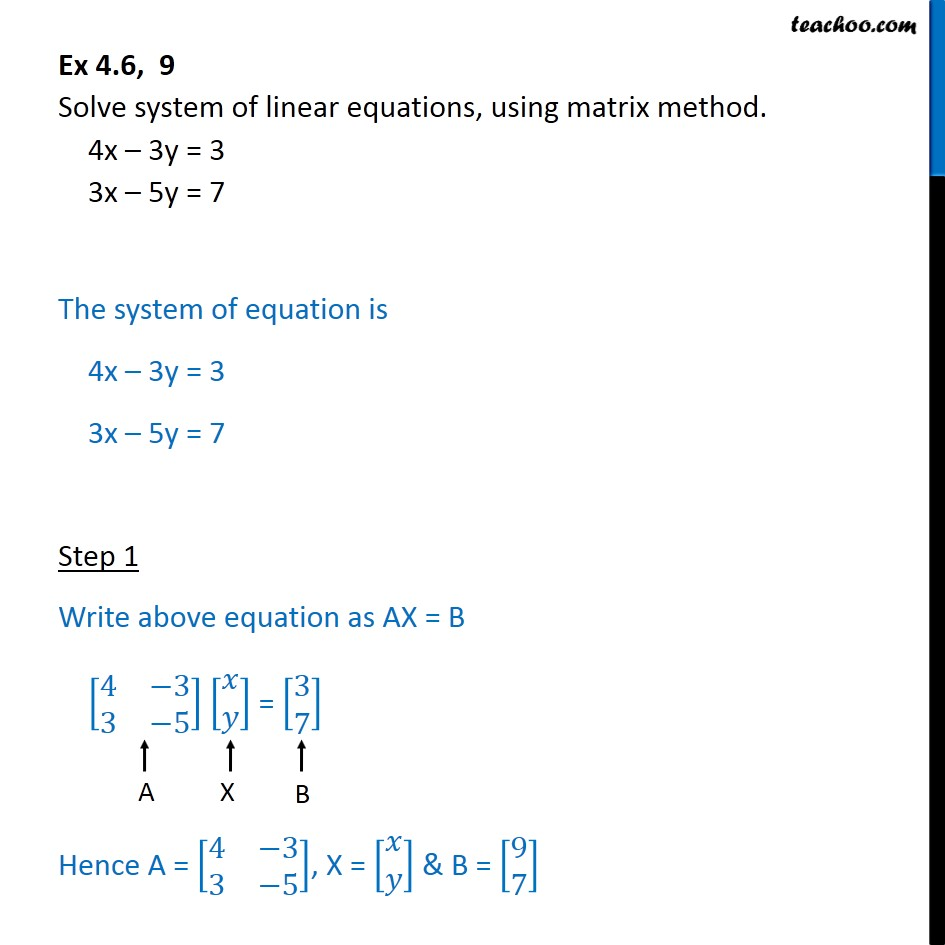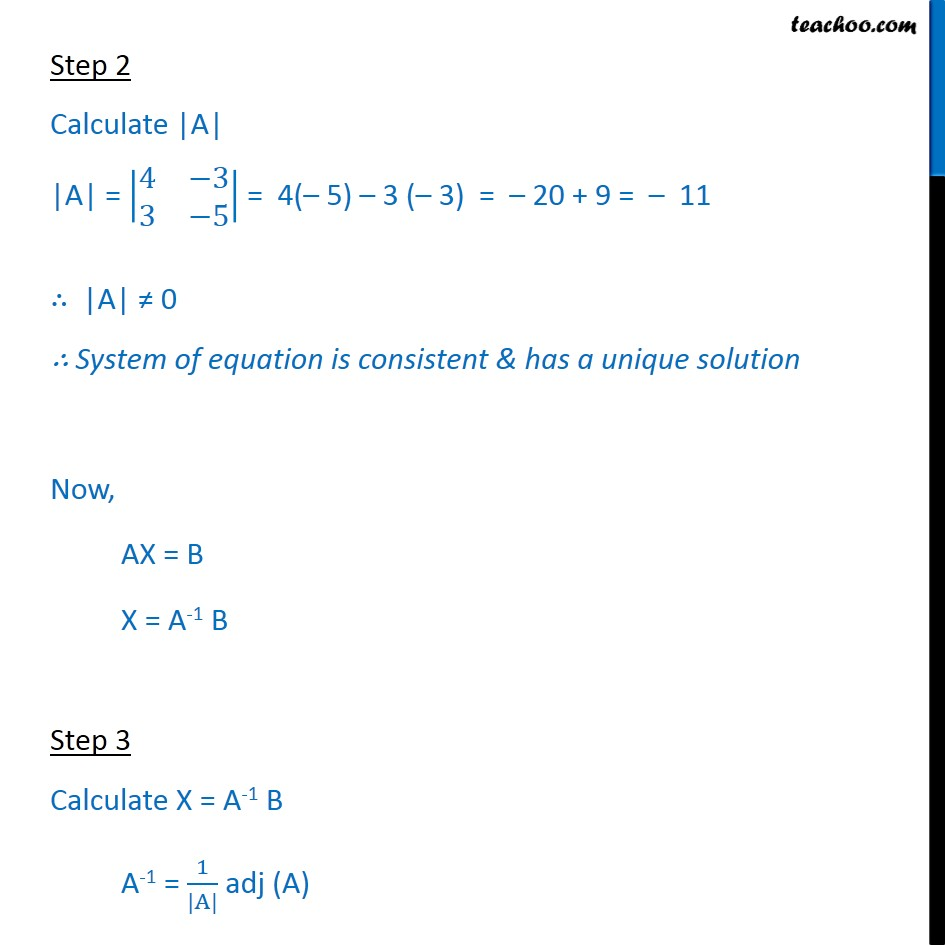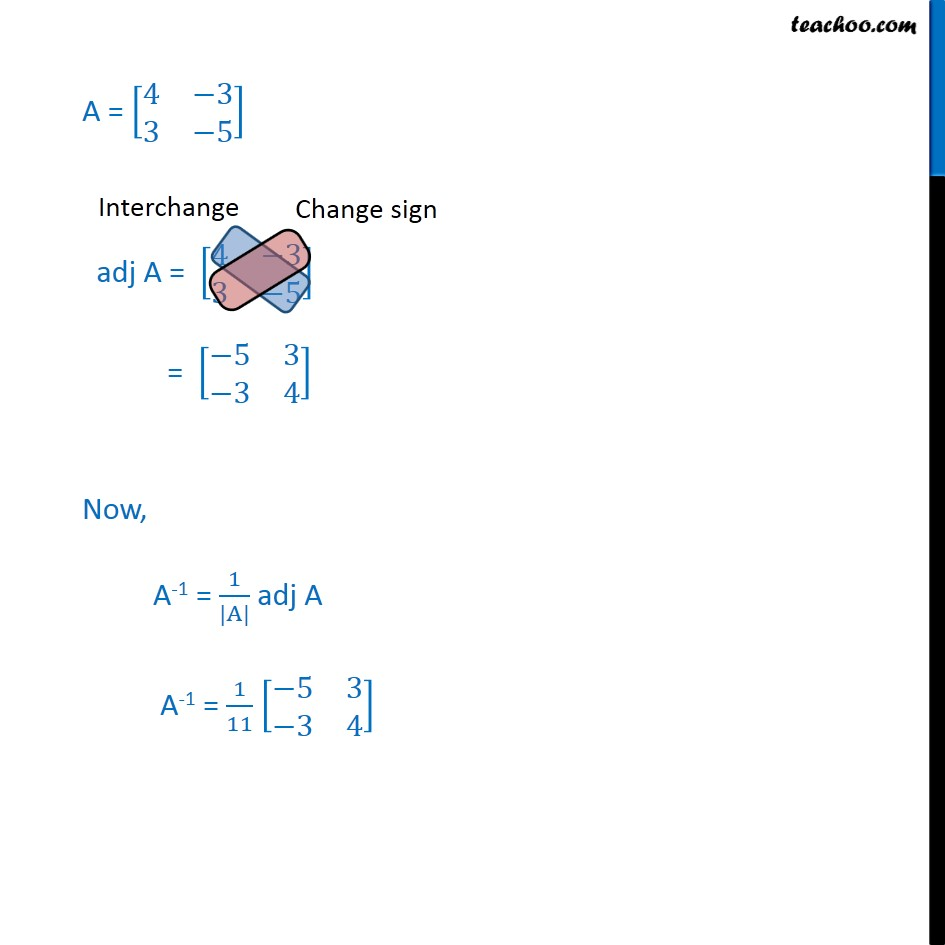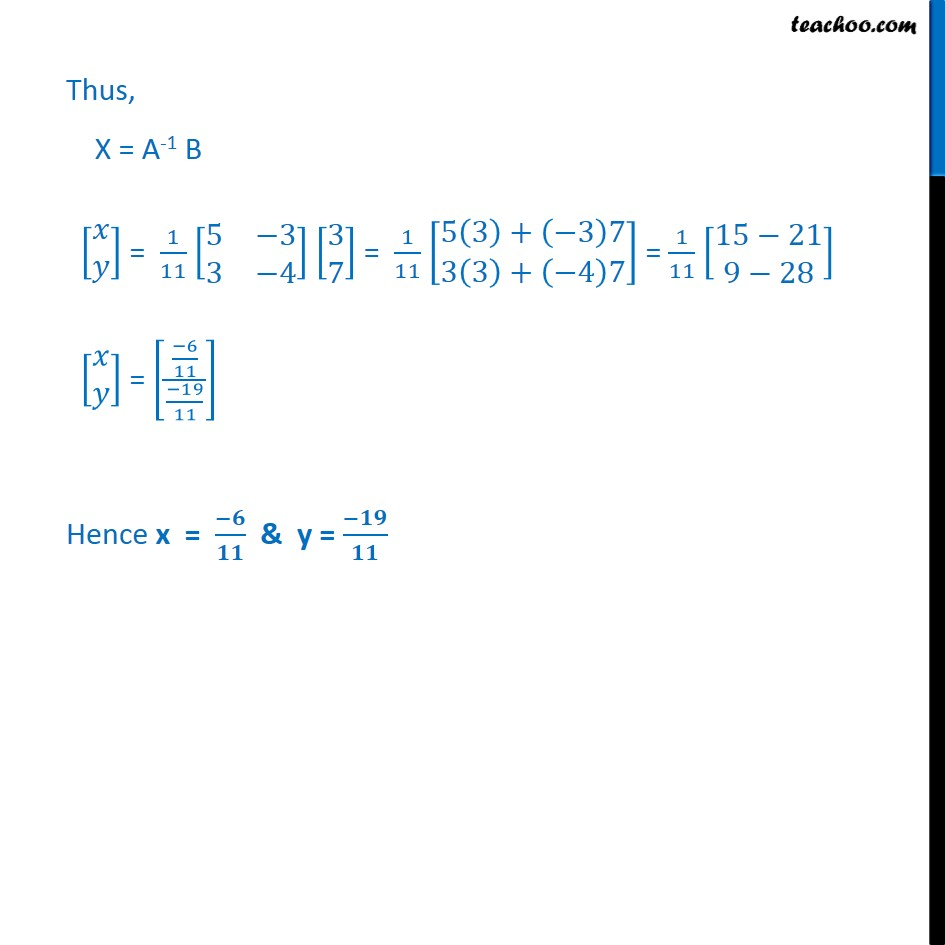1. Chapter 4 Class 12 Determinants
2. Serial order wise
3. Ex 4.6

Transcript

Ex 4.6, 9 Step 2 Calculate |A| |A| = 4 3 3 5 = 4( 5) 3 ( 3) = 20 + 9 = 11 |A| 0 System of equation is consistent & has a unique solution Now, AX = B X = A-1 B Step 3 Calculate X = A-1 B A-1 = 1 |A| adj (A) A = 4 3 3 5 adj A = 4 3 3 5 = 5 3 3 4 Now, A-1 = 1 |A| adj A A-1 = 1 11 5 3 3 4 Thus, X = A-1 B = 1 11 5 3 3 4 3 7 = 1 11 5 3 + 3 7 3 3 + 4 7 = 1 11 15 21 9 28 = 6 11 19 11 Hence x = & y =

Ex 4.6